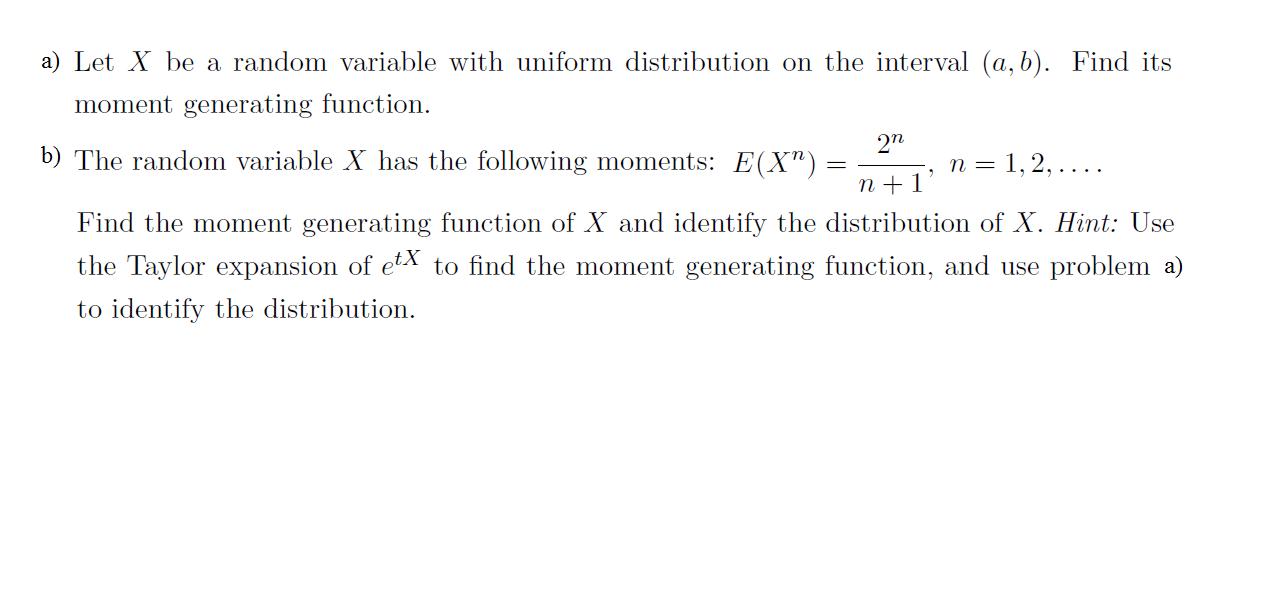# a) Let Xbe a random variable with uniform distribution on the interval (a, b). Find itsmoment generating function272b) The random variable X has the following moments: E(X")n =1,2,... .n1Find the moment generating function of X and identify the distribution of X. Hint: Usethe Taylor expansion of etX to find the moment generating function, and use problem a)to identify the distribution

Question

Escalate - Show every mynute steps in DETAILED!help_outlineImage Transcriptionclosea) Let Xbe a random variable with uniform distribution on the interval (a, b). Find its moment generating function 272 b) The random variable X has the following moments: E(X") n =1,2,... . n1 Find the moment generating function of X and identify the distribution of X. Hint: Use the Taylor expansion of etX to find the moment generating function, and use problem a) to identify the distribution fullscreen
check_circleExpert Solution
Step 1

As you have posted 2 different questions, we are answering the first question. In case you require the unanswered questions also, kindly re-post them as separate questions.

Step 2

a)

From the given information, the random variable X follows uniform distribution on interval

(a, b).

The probability density function for X is,

Step 3

The moment generating...

### Want to see the full answer?

See Solution

#### Want to see this answer and more?

Solutions are written by subject experts who are available 24/7. Questions are typically answered within 1 hour*

See Solution
*Response times may vary by subject and question
Tagged in

### Other